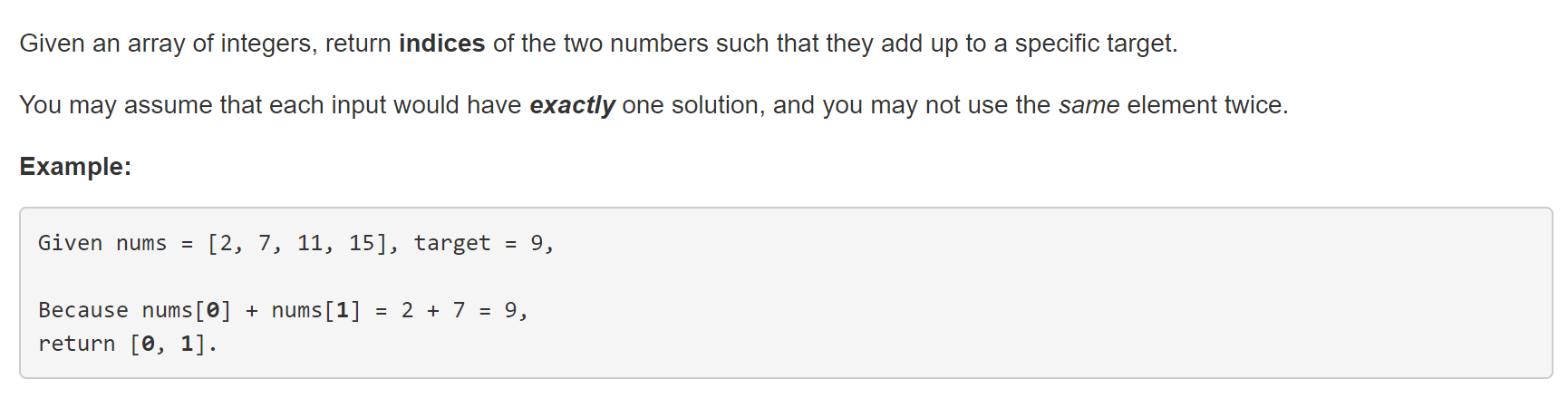# 从TwoSum浅析时间复杂度的优化策略

TwoSumclass Solution {
public:
vector<int> twoSum(vector<int>& nums, int target) {
for (int i = 0; i < nums.size(); i++)//用二重循环进行查找，时间复杂度为O(n^2)
{
for (int j = i + 1; j < nums.size(); j++)
{
if (nums[i] + nums[j] == target)
{
vector<int> vec(2);
vec = i;
vec = j;
return vec;
}
}
}
}
};

（1）使用二重循环来遍历整个数组是没有必要的，凭空增加了不必要的时间复杂度。
（2）过于简单和不动脑筋，查找还有很多其他的方法可以使用，而非只有遍历。

static int x = [](){
std::ios::sync_with_stdio(false);
cin.tie(NULL);
return 0;
}();

class Solution {
public:
vector<int> twoSum(vector<int>& nums, int target) {
vector<int> result;
map<int,int> numNeeded;
std::map<int,int>::iterator it;

int i,targNum, len = nums.size();

for(i = 0; i < len; i++)
{
it = numNeeded.find(nums[i]);
if(it == numNeeded.end())
{
targNum = target - nums[i];
numNeeded.insert(numNeeded.begin(), std::pair<int,int>(targNum,i));
}
else
{
return vector<int> {it->second, i};
}
}
return result;
}
};

cin.tie与sync_with_stdio加速输入输出

sync_with_stdio这个函数是一个“是否兼容stdio”的开关，C++为了兼容C，保证程序在使用了std::printf和std::cout的时候不发生混乱，将输出流绑到了一起。

3ms的代码中，加快查找速度主要是依靠c++中的关联式容器map。